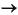ProgrammingConverting from String to Numeric

The functions listed in this table provide a number of ways to convert character strings to numeric data.

 Function Description Example `uintN`(e.g., `uint8)` Convert a character to an integer code that represents that character. `'Hi'``72 105` `str2num` Convert a character type to a numeric type. `'72 105'``[72 105]` `str2double` Similar to `str2num`, but offers better performance and works with cell arrays of strings. `{'72' '105'}`` [72 105]` `hex2num` Convert a numeric type to a character type of specified precision, returning a string that MATLAB can evaluate. `'A'``'-1.4917e-154'` `hex2dec` Convert a character type of hexadecimal base to a positive integer. `'A'``10` `bin2dec` Convert a positive integer to a character type of binary base. `'1010'``10` `base2dec` Convert a positive integer to a character type of any base from 2 through 36. `'12'``10`(if `base` == `8`)

Converting from a Character Equivalent

Character arrays store each character as a 16-bit numeric value. Use one of the integer conversion functions (e.g., `uint8`) or the `double` function to convert strings to their numeric values, and `char` to revert to character representation:

• ```name = 'Thomas R. Lee';

name = double(name)
name =
84  104  111  109  97  115  32  82  46  32  76  101  101

name = char(name)
name =
Thomas R. Lee
```

Converting from a Numeric String

Use `str2num` to convert a character array to the numeric value represented by that string:

• ```str = '37.294e-1';

val = str2num(str)
val =
3.7294
```

The `str2double` function converts a cell array of strings to the double-precision values represented by the strings:

• ```c = {'37.294e-1'; '-58.375'; '13.796'};

d = str2double(c)
d =
3.7294
-58.3750
13.7960

whos
Name      Size                   Bytes  Class

c         3x1                      224  cell array
d         3x1                       24  double array
```

Converting from a Specific Radix

To convert from a character representation of a nondecimal number to the value of that number, use one of these functions: `hex2num`, `hex2dec`, `bin2dec`, or `base2dec`.

The `hex2num` and `hex2dec` functions both take hexadecimal (base 16) inputs, but `hex2num` returns the IEEE double-precision floating-point number it represents while `hex2dec` converts to a decimal integer.Converting from Numeric to String Function Summary© 1994-2005 The MathWorks, Inc.